Search by Topic

Resources tagged with Complex numbers similar to Complex Sine:

Filter by: Content type:
Age range:
Challenge level:

There are 21 results

Broad Topics > Numbers and the Number System > Complex numbersComplex Sine

Age 16 to 18 Challenge Level:

Solve the equation sin z = 2 for complex z. You only need the formula you are given for sin z in terms of the exponential function, and to solve a quadratic equation and use the logarithmic function.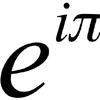An Introduction to Complex Numbers

Age 16 to 18

A short introduction to complex numbers written primarily for students aged 14 to 19.Footprints

Age 16 to 18 Challenge Level:

Make a footprint pattern using only reflections.Two and Four Dimensional Numbers

Age 16 to 18 Challenge Level:

Investigate matrix models for complex numbers and quaternions.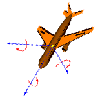Complex Rotations

Age 16 to 18 Challenge Level:

Choose some complex numbers and mark them by points on a graph. Multiply your numbers by i once, twice, three times, four times, ..., n times? What happens?Sheep in Wolf's Clothing

Age 16 to 18 Challenge Level:

Can you work out what simple structures have been dressed up in these advanced mathematical representations?Roots and Coefficients

Age 16 to 18 Challenge Level:

If xyz = 1 and x+y+z =1/x + 1/y + 1/z show that at least one of these numbers must be 1. Now for the complexity! When are the other numbers real and when are they complex?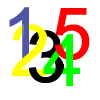Complex Countdown

Age 16 to 18 Challenge Level:

Play a more cerebral countdown using complex numbers.What Are Numbers?

Age 7 to 18

Ranging from kindergarten mathematics to the fringe of research this informal article paints the big picture of number in a non technical way suitable for primary teachers and older students.Pumping the Power

Age 16 to 18 Challenge Level:

What is an AC voltage? How much power does an AC power source supply?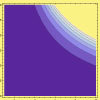Sextet

Age 16 to 18 Challenge Level:

Investigate x to the power n plus 1 over x to the power n when x plus 1 over x equals 1.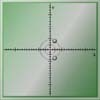Conjugate Tracker

Age 16 to 18 Challenge Level:

Make a conjecture about the curved track taken by the complex roots of a quadratic equation and use complex conjugates to prove your conjecture.Napoleon's Theorem

Age 14 to 18 Challenge Level:

Triangle ABC has equilateral triangles drawn on its edges. Points P, Q and R are the centres of the equilateral triangles. What can you prove about the triangle PQR?What Are Complex Numbers?

Age 16 to 18

This article introduces complex numbers, brings together into one bigger 'picture' some closely related elementary ideas like vectors and the exponential and trigonometric functions and. . . .8 Methods for Three by One

Age 14 to 18 Challenge Level:

This problem in geometry has been solved in no less than EIGHT ways by a pair of students. How would you solve it? How many of their solutions can you follow? How are they the same or different?. . . .Three by One

Age 16 to 18 Challenge Level:

There are many different methods to solve this geometrical problem - how many can you find?Impedance Can Be Complex!

Age 16 to 18 Challenge Level:

Put your complex numbers and calculus to the test with this impedance calculation.Thousand Words

Age 16 to 18 Challenge Level:

Here the diagram says it all. Can you find the diagram?Complex Partial Fractions

Age 16 to 18 Challenge Level:

To break down an algebraic fraction into partial fractions in which all the denominators are linear and all the numerators are constants you sometimes need complex numbers.Cube Roots

Age 16 to 18 Challenge Level:

Evaluate without a calculator: (5 sqrt2 + 7)^{1/3} - (5 sqrt2 - 7)^1/3}.Target Six

Age 16 to 18 Challenge Level:

Show that x = 1 is a solution of the equation x^(3/2) - 8x^(-3/2) = 7 and find all other solutions.